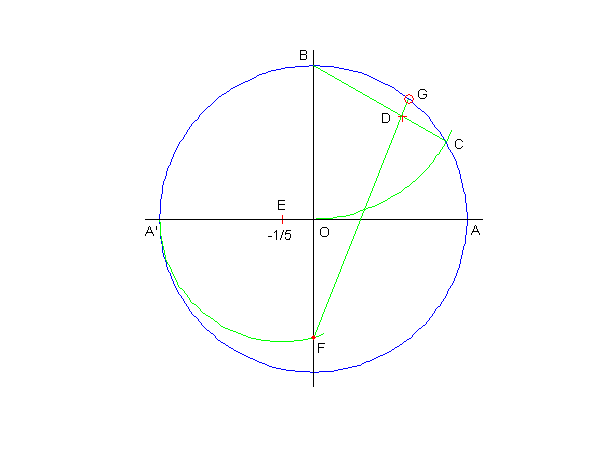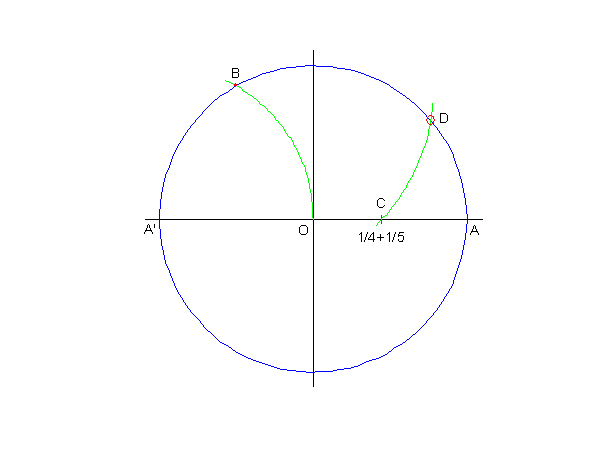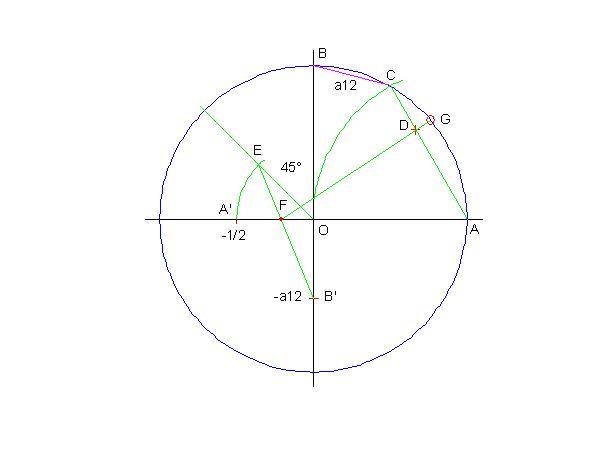# Approximate Construction of Heptagon and Nonagon

Avni Pllana

A simple approximate construction of heptagon is shown in Fig.1.Fig.1

First we construct points C = 1/2 and D = 1/5 on the x-axis of the unit circle, as shown in Fig.1. Then we construct point E = 1/2( C + D ). The line BE intersects the circle at point F. Now we have

angle AOF = 51.4199° .

The angle subtended by a side of a regular heptagon is 360° / 7 = 51.4286° . So angle AOF is about 0.0087° off, which can be considered as a good approximation.

Another more accurate construction of heptagon is shown in Fig.2.Fig.2

The arc with center at B and radius OA = 1 intersects the unit circle at point C. On the line BC we construct point D such that CD = 1/3CB. The arc with center at E = -1/5 and radius EA’ intersects the y-axis of the unit circle at point F. The line FD intersects the circle at point G. Now we have

angle AOG = 51.4289° ,

which can be considered as a very good approximation.

An approximate construction of nonagon is shown in Fig.3.Fig.3

The arc with center at A’ and radius OA = 1 intersects the unit circle at point B. We construct point C such that C = 1/4 +1/5. The arc with center at B and radius BC intersects the circle at point D. Now we have

angle AOD = 40.0059° .

The angle subtended by a side of a regular nonagon is 360° / 9 = 40° , so angle AOD can be considered as a good approximation.

Another more accurate construction of nonagon is shown in Fig.4.Fig.4

The arc with center at A and radius OA = 1 intersects the unit circle at point C. On the line AC we construct point D such that CD = 1/3CA. The arc with center at O and radius OA’ = 1/2 intersects the bisector of the second quadrant at point E. On the negative y-axis we construct point B’ = -a12, where a12 is the side of the regular 12-gon (dodecagon). The line B’E intersects the x-axis at point F. The line FD intersects the circle at point G. Now we have

angle AOG = 40.0002° ,

which can be considered as a very good approximation.

Hosted by www.Geocities.ws